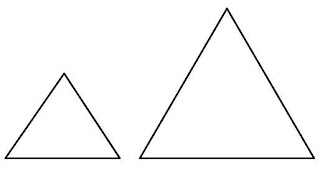## Tuesday, November 29, 2016

### Geometry : Harder Chapter Level QuesitonsQuestion #1 : The area ratio of two equilateral triangles are 4 to 9 and the sum of their perimeter is 30 3 . What is the area of the a. smaller triangle   b. larger triangle?

Solution:
If the area ratio of two similar polygons is 4 to 9, their corresponding line ratio would be  4  to  9
or 2 to 3.[Make sure you know why.]
The perimeter of the two equilateral triangles is 30 3 so the smaller triangle has a perimeter of
2/5 *  30 3 or 12 3. One side is 4 3 . Using the formula of finding the area of an equilateral triangle $$\dfrac{\sqrt3*s^2}{4}$$ , you get the area to be 12 3.

Use the same method to get the area of the larger triangle as 27 3.
You can also use ratio relationship to get the area of the larger triangle by
multiply 12 3 by 9/4.

2007 Mathcounts Chapter Sprint #30: In parallelogram ABCD, AB = 16 cm, DA = 32 cm, and sides AB and DA form a 45-degree interior angle. In isosceles trapezoid WXYZ with WX ≠ YZ, segment WX is the longer parallel side and has length 16 cm, and two interior angles each have a measure of 45 degrees. Trapezoid WXYZ has the same area as parallelogram ABCD. What is the length of segment YZ?

Solution I:
Make sure you know how to get the unknown leg fast. The height of the parallelogram is 82, so the area of the parallelogram is 48 square units. [Check out the special right triangle section here if you can't get the height fast.]

Let YZ of the trapezoid be x and draw the height. Using 45-45-90 degree angle ratio, you'll get the height. (See image above.)
Area of the trapezoid is average of the two bases time height. WX = 16 (given)
$$\dfrac{(16+x)* (16-x)}{4}$$ = 48 ; 256 - x2 = 192 ;  - x2 = - 64;  x = 8 = YZ

Solution II:

Make the y be the height of the trapezoid. YZ = 16 - 2y.  $$\dfrac{(16-2y + 16)}{2}$$ * y = 48
$${(16 -y)* y = 48}$$$$\rightarrow$$ $${16y -y^2 = 48}$$ $$\rightarrow$$ $${y^2 - 16y + 48 = 0}$$ $$\rightarrow$$ $${(y -12)(y -4)=0}$$ $$\rightarrow$$ $${y = 4}$$ or $${y = 12}$$(doesn't work)
YZ = 16 - 2y. Plug in y = 4 and you have  YZ = 8

Solution III: Let the height be y and you have $$\dfrac{(\overline{YZ}+ 16)* y}{2}"$$ = 48 ; ( YZ + 16) * y = 96
When there are some numbers multiply together equal another number, it's a factoring question.
32 * 3 = 96, YZ = 16 (doesn't work)
24 * 4 = 96, YZ =8